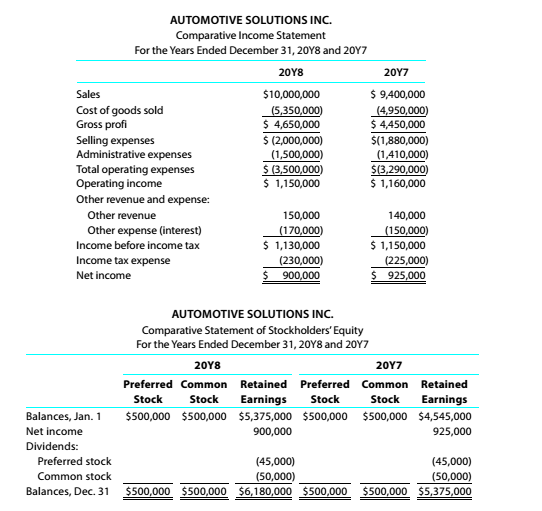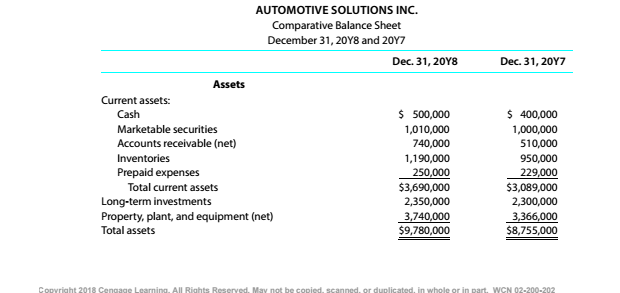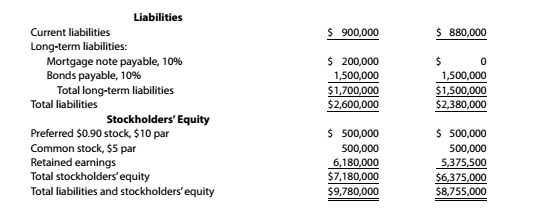Chapter 9, Problem 9.4.11P

Chapter
Section
Textbook Problem

Twenty metrics of liquidity, solvency, and profitabilityThe comparative financial statements of Automotive Solutions Inc. are as follows. The market price of Automotive Solutions Inc. common stock was \$119.70 on December 31, 20Y8InstuctionsTunes earned

To determine

Concept Introduction:

Times interest earned Ratio:

A company pays its interest expenses from the Net operating Income available. To find the company's ability to pay the interest expenses, the ratio of Net operating income to interest expenses should be calculated. Interest rate earned ratio shows the number of times interest expenses are covered by the net operating income. It is calculated by dividing the Net operating Income by interest expenses. The formula is as under:

interest earned Ratio = Net Operating Income / interest expenses

(Note: The numerator of the formula "Net operating income" is equal to the Income before deduction of Interest and taxes)

To Calculate:

The internet earned.

Explanation

The Times interest earned is calculated as follows:

 20Y8 20Y7 Income before taxes (A) \$ 1,130,000 \$ 1,150,000 Add: Interest Expense (B)

Still sussing out bartleby?

Check out a sample textbook solution.

See a sample solution

The Solution to Your Study Problems

Bartleby provides explanations to thousands of textbook problems written by our experts, many with advanced degrees!

Get Started

Find more solutions based on key concepts